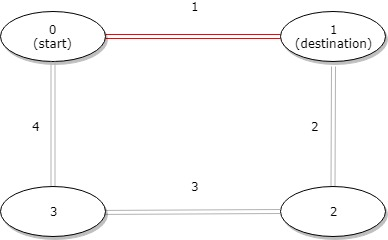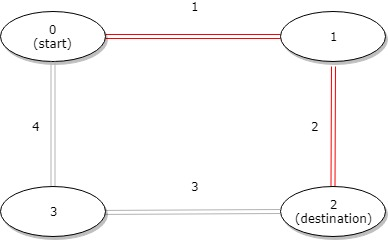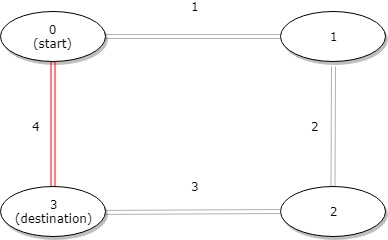#DAY 7
0
Software Development

## 【Day 7】#1184 - Distance Between Bus Stops

A bus has n stops numbered from 0 to n - 1 that form a circle. We know the distance between all pairs of neighboring stops where distance[i] is the distance between the stops number i and (i + 1) % n.

The bus goes along both directions i.e. clockwise and counterclockwise.

Return the shortest distance between the given start and destination stops.

Example 1:``````Input: distance = [1,2,3,4], start = 0, destination = 1
Output: 1
Explanation: Distance between 0 and 1 is 1 or 9, minimum is 1.
``````

Example 2:``````Input: distance = [1,2,3,4], start = 0, destination = 2
Output: 3
Explanation: Distance between 0 and 2 is 3 or 7, minimum is 3.
``````

Example 3:``````Input: distance = [1,2,3,4], start = 0, destination = 3
Output: 4
Explanation: Distance between 0 and 3 is 6 or 4, minimum is 4.
``````

Constraints:

• 1 <= n <= 10^4
• distance.length == n
• 0 <= start, destination < n
• 0 <= distance[i] <= 10^4

## 解法

``````class Solution {
public int distanceBetweenBusStops(int[] distance, int start, int destination) {
if (start == destination) {
return 0;
}
int clockwiseSum = 0, counterclockwiseSum = 0, begin = start, end = destination;

// Calculate the distance clockwise
if (destination % distance.length < start % distance.length) {
// To know how many stops between start and destination
end += distance.length;
}
for (int i = begin; i < end; i++) {
int busStop = i % distance.length;
clockwiseSum += distance[busStop];
}

// Calculate the distance counterclockwise
begin = start;
end = destination;
if (destination % distance.length > start % distance.length) {
// To know how many stops between start and destination
begin += distance.length;
}
for (int i = begin - 1; i >= end; i--) {
int busStop = i % distance.length;
counterclockwiseSum += distance[busStop];
}

// Compare both distances to find out the shortest distance
if (clockwiseSum < counterclockwiseSum) {
return clockwiseSum;
} else {
return counterclockwiseSum;
}
}
}
``````

LeetCode小試身手14